# Linear Equations in Two Variables (Mathematics) Class 9 - NCERT Questions

Q 1.

The cost of a notebook is twice the cost of a pen. Write a linear equation in two variables to represent this statement. (Take the cost of a notebook to be ₹x and that of a pen to be₹ y).

SOLUTION:

Let the cost of a note book = ₹x
and the cost of a pen = ₹ y
According to the condition, we have
[Cost of a notebook] = 2 x [Cost of a pen]
[Cost of a notebook] = 2 x [Cost of a pen]
i.e. (x) = 2 x (y) or, x = 2y
or, x - 2y = 0
Thus, the required linear equation is x - 2y = 0.

Q 2.

Express the following linear equations in the form ax + by + c = 0 and indicate the values of a, b and c in each case.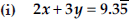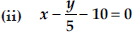(iii) -2x + 3y = 6 (iv) x = 3y
(v) 2x = - 5y (vi) 3x + 2 = 0
(vii) y - 2 = 0 (viii) 5 = 2x

SOLUTION:

(i) We have 2x + 3y =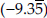or (2)x + (3)y += 0
Comparing it with ax + by + c = 0, we get a = 2, b = 3 and c =(ii) We have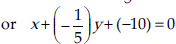Comparing it with ax + by + c = 0, we get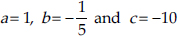(iii) We have -2x + 3y = 6
or (-2)x + (3)y + (-6) = 0
Comparing it with ax + by + c = 0, we get a = - 2, b = 3 and c = - 6.
(iv) We have x = 3y or (1)x + (-3)y + (0) = 0
Comparing it with ax + by + c = 0, we get a = 1, b = -3 and c = 0.
(v) We have 2x = -5y or (2)x + (5)y + (0) = 0
Comparing it with ax + by + c = 0, we get a = 2, b = 5 and c = 0.
(vi) We have 3x + 2 = 0
or (3)x + (0)y + (2) = 0
Comparing it with ax + by + c = 0, we get a = 3, b = 0 and c = 2.
(vii) We have y - 2 = 0
or (0)x + (1)y + (-2) = 0
Comparing it with ax + by + c = 0, we get a = 0, b = 1 and c = -2.
(viii) We have 5 = 2x
⇒ 5 - 2x = 0 ⇒ - 2x + 0y + 5 = 0
⇒ (-2)x + (0)y + (5) = 0
Comparing it with ax + by + c = 0, we get a = -2, b = 0 and c = 5.

Q 3.

Which one of the following options is true, and why?
y = 3x + 5 has
(i) a unique solution
(ii) only two solutions
(iii) infinitely many solutions

SOLUTION:

Option (iii) is true because a linear equation has an infinitely many solutions. Moreover when represented graphically a linear equation in two variables is a straight line which has infinite points and
hence, it has infinite solutions.

Q 4.

Write four solutions for each of the following equations:
(i) 2x + y = 7 (ii) px + y = 9 (iii) x = 4y

SOLUTION:

(i) 2x + y = 7
When x = 0, 2(0) + y = 7
⇒ 0 + y = 7 ⇒ y = 7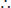Solution is (0, 7)
When x = 1, 2(1) + y = 7
⇒ y = 7 - 2 ⇒ y = 5Solution is (1, 5)
When x = 2, 2(2) + y = 7
⇒ y = 7 - 4 ⇒ y = 3Solution is (2, 3)
When x = 3, 2(3) + y = 7
⇒ y = 7 - 6 ⇒ y = 1Solution is (3, 1).
(ii) px + y = 9
When x = 0, p(0) + y = 9
⇒ y = 9 - 0 ⇒ y = 9Solution is (0, 9)
When x = 1, p(1) + y = 9
⇒ y = 9 - pSolution is (1, (9 -p))
When x = 2, p(2) + y = 9 ⇒ y = 9 - 2pSolution is (2, (9 - 2p))
When x = -1, p(-1) + y = 9 ⇒ -p + y = 9
⇒ y = 9 + pSolution is (-1, (9 + p))
(iii) x = 4y
When x = 0, 4y = 0 ⇒ y = 0Solution is (0, 0)
When x = 1, 4y = 1Solution is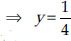When x = 4, 4 = 4y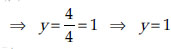Solution is (4, 1)
When x = -4, 4y = -4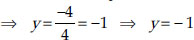Solution is (-4, -1).

Q 5.

Check which of the following are solutions of the equation x - 2y = 4 and which are not: (i) (0, 2) (ii) (2, 0)
(iii) (4, 0)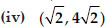(v) (1, 1)

SOLUTION:

(i) (0, 2) means x = 0 and y = 2
Putting x = 0 and y = 2 in x - 2y = 4, we have
L.H.S. = 0 - 2(2) = - 4. But R.H.S. = 4L.H.S. ≠ R.H.S.x = 0, y = 2 is not a solution.
(ii) (2, 0) means x = 2 and y = 0
Putting x = 2 and y = 0 in x - 2y = 4, we get
L.H.S. = 2 - 2(0) = 2 - 0 = 2. But R.H.S. = 4L.H.S. ≠ R.H.S.(2, 0) is not a solution.
(iii) (4, 0) means x = 4 and y = 0
Putting x = 4 and y = 0 in x - 2y = 4, we get
L.H.S. = 4 - 2(0) = 4 - 0 = 4 = R.H.S.L.H.S. = R.H.S.(4, 0) is a solution.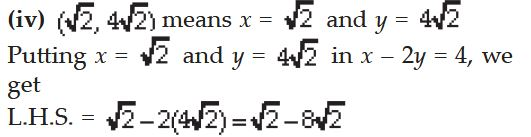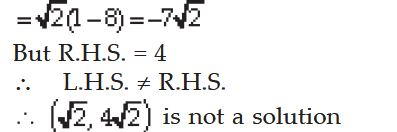(v) (1, 1) means x = 1 and y = 1
Putting x = 1 and y = 1 in x - 2y = 4, we get
L.H.S. = 1 - 2(1) = 1 - 2 = -1. But R.H.S. = 4L.H.S. ≠ R.H.S.(1, 1) is not a solution.

Q 6.

Find the value of k, if x = 2, y = 1 is a solution of the equation 2x + 3y = k

SOLUTION:

We have 2x + 3y = k
Putting x = 2 and y = 1 in 2x + 3y = k, we get 2(2) + 3(1) = k
⇒ 4 + 3 = k ⇒ 7 = k
Thus, the required value of k = 7.

Q 7.

Draw the graph of each of the following linear equations in two variables:
(i) x + y = 4 (ii) x - y = 2
(iii) y = 3x (iv) 3 = 2x + y

SOLUTION:

(i) x + y = 4 ⇒ y = 4 - x
If we have x = 0, then y = 4 - 0 = 4
x = 1, then y = 4 - 1 = 3
x = 2, then y = 4 - 2 = 2We get the following table :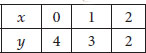Plot the ordered pairs (0, 4), (1, 3) and (2, 2) on the graph paper. Joining these points, we get a line AB as shown.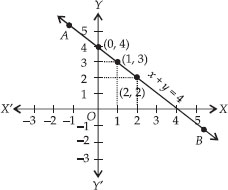Thus, the line AB is the required graph of x + y = 4
(ii) x - y = 2 ⇒ y = x - 2
If we have x = 0, then y = 0 - 2 = -2
x = 1, then y = 1 - 2 = -1
x = 2, then y = 2 - 2 = 0We have the following table :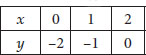Plot the ordered pairs (0, -2), (1, -1) and (2, 0) on the graph paper. Joining these points, we get a straight line PQ as shown.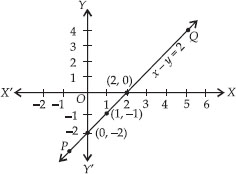Thus, the line PQ is required graph of x - y = 2
(iii) y = 3x
If we have x = 0, then y = 3(0) ⇒ y = 0
x = 1, then y = 3(1) ⇒ y = 3
x = -1, then y = 3(-1) ⇒ y = -3We get the following table: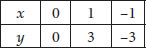Plot the ordered pairs (0, 0), (1, 3) and (-1, -3) on the graph paper. Joining these points, we get a straight line LM.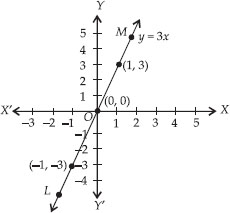Thus, LM is the required graph of y = 3x.
(iv) 3 = 2x + y ⇒ y = 3 - 2x
If we have x = 0, then y = 3 - 2(0) ⇒ y = 3
x = 1, then y = 3 - 2(1) ⇒ y = 1
x = 2, then y = 3 - 2(2) = 3 - 4 = - 1
⇒ y = - 1We get the following table :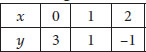Plot the ordered pairs (0, 3), (1, 1) and (2, - 1) on the graph paper. Joining these points, we get a line CD.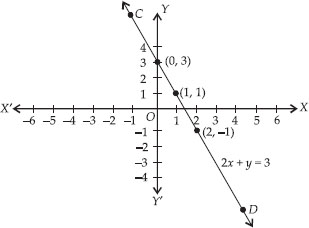Thus, the line CD is the required graph of 3 = 2x + y.

Q 8.

Give the equations of two lines passing through (2, 14). How many more such lines are there, and why?

SOLUTION:

(2, 14) means x = 2 and y = 14
Following equations can have (2, 14) as the solution, i.e. they can pass through the point (2, 14).
(i) x + y = 16
(ii) 7x - y = 0
There are infinite number of lines which passes through the point (2, 14), because infinite number of lines can pass through a point

Q 9.

If the point (3, 4) lies on the graph of the equation 3y = ax + 7, find the value of a.

SOLUTION:

The equation of the given line is 3y = ax + 7
As,(3, 4) lies on the given line
it must satisfy the equation 3y = ax + 7
We have (3, 4) ⇒ x = 3 and y = 4, putting these values in equation, we get
3 x 4 = a x 3 + 7 ⇒ 12 = 3a + 7
⇒ 3a = 12 - 7 = 5 Therefore,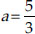Thus, the required value of a is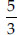Q 10.

The taxi fare in a city is as follows :
For the first kilometre, the fare is ₹ 8 and for the subsequent distance it is ₹ 5 per km. Taking the distance covered as x km and total fare as ₹ y, write a linear equation for this information and draw its graph.

SOLUTION:

Here, total distance covered = x km and total taxi fare = ₹y
Fare for the lst km = ₹8
Remaining distance = (x - 1) km
Therefore, Fare for (x - 1) km = ₹ 5 x (x - 1)
Total taxi fare = ₹ 8 + ₹ 5(x - 1)
According to the condition,
y = 8 + 5(x - 1) ⇒ y = 8 + 5x - 5 ⇒ y = 5x + 3
Which is the required linear equation representing the given information.
Graph : We have y = 5x + 3
Therefore, When x = 0, then y = 5(0) + 3 ⇒ y = 3
x = - 1, then y = 5(-1) + 3 ⇒ y = -2
x = -2, then y = 5(-2) + 3 ⇒ y = -7
Therefore, We get the following table: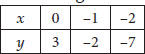Now, plotting the ordered pairs (0, 3), (-1, -2) and (-2, -7) on a graph paper and joining them, we get a straight line PQ. Thus, PQ is the required graph of the linear equati on y = 5x + 3

Q 11.

From the choices given below, choose the equation whose graphs are given in Fig. (1) and Fig. (2).
For Fig. (1)
(i) y = x
(ii) x + y = 0
(iii) y = 2x
(iv) 2 + 3y = 7x
For Fig. (2)
(i) y = x + 2
(ii) y = x - 2
(iii) y = -x + 2
(iv) x + 2y = 6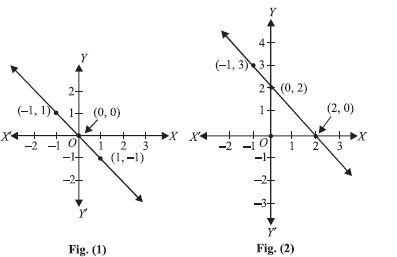SOLUTION:

For Fig. (1), the correct linear equation is x + y = 0
[As (-1, 1) ⇒ -1 + 1 = 0
and (1, -1) ⇒ 1 + (-1) = 0]
For Fig. (2), the correct linear equation is y = -x + 2
[As (-1, 3) ⇒ 3 = -1(-1) + 2 ⇒ 3 = 3 and (0, 2) ⇒ 2 = -(0) + 2 ⇒ 2 = 2]

Q 12.

If the work done by a body on application of a constant force is directly proportional to the distance travelled by the body, express this in the form of an equation in two variables and draw the
graph of the same by taking the constant force as 5 units. Also read from the graph the work done when the distance travelled by the body is
(i) 2 units (ii) 0 unit

SOLUTION:

Constant force is 5 units. Let the distance travelled = x units and work done = y units.
Since, Work done = Force x Displacement
⇒ y = 5 x x ⇒ y = 5x
For drawing the graph, we have y = 5x
When x = 0, then y = 5(0) = 0
x = 1, then y = 5(1) = 5
x = -1, then y = 5(-1) = -5
Therefore, We get the following table: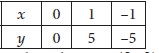Plotting the ordered pairs (0, 0), (1, 5) and (-1, -5) on the graph paper and joining the points, we get a straight line AB.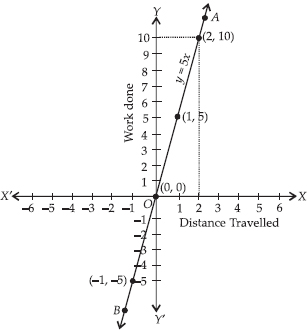From the graph, we get
(i) Distance travelled = 2 units i.e., x = 2
If x = 2, then y = 10 units
⇒ Work done = 10 units.
(ii) Distance travelled = 0 unit i.e., x = 0
If x = 0 ⇒ y = 5(0) = 0
⇒ Work done = 0 unit.

Q 13.

Yamini and Fatima, two students of Class IX of a school, together contributed ' 100 towards the Prime Minister’s Relief Fund to help the earthquake victims. Write a linear equation
which satisfies this data. (You may take their contributions as ' x and ' y.) Draw the graph of the same.

SOLUTION:

Let the contribution of Yamini = ₹ x and the contribution of Fatima = ₹ yWe have x + y = 100 ⇒ y = 100 - x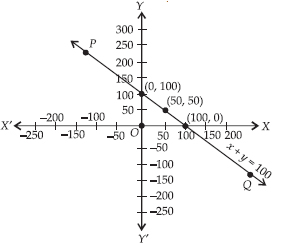Now, when x = 0, y = 100 - 0 = 100 x = 50, y = 100 - 50 = 50 x = 100, y = 100 - 100 = 0We get the following table: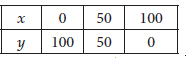Plotting the ordered pairs (0, 100), (50, 50) and (100, 0) on a graph paper and using proper scale and joining these points we get a line PQ.

Q 14.

In countries like USA and Canada, temperature is measured in Fahrenheit, whereas in countries like India, it is measured in Celsius. Here is a linear equation that converts Fahrenheit to
Celsius.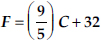(i) Draw the graph of the linear equation above using Celsius for x-axis and Fahrenheit for y-axis.
(ii) If the temperature is 30°C, what is the temperature in Fahrenheit?
(iii) If the temperature 95°F, what is the temperature in Celsius?
(iv) If the temperature is 0°C, what is the temperature in Fahrenheit and if the temperature is 0°F, what is the temperature in Celsius?
(v) Is there a temperature which is numerically the same in both Fahrenheit and Celsius ? If yes, find it.

SOLUTION:

(i) We haveWhen C = 0,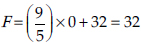When C = -15,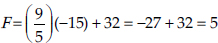When C = - 10,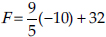= - 18 + 32 = 14
We have the following table: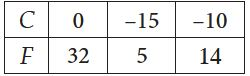Plotting the ordered pairs (0, 32), (-15, 5) and (-10, 14) on a graph paper. Joining these points we get straight line AB.
(ii) From the graph, we have 86°F corresponds to 30°C
(iii) From the graph, we have 95°F = 35°C
(iv) From the graph, we have 0°C = 32°F and 0°F = - 17.8°C
(v) When F = C (numerically)
From given equation, we get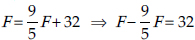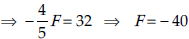Temperature is - 40° both in F and C.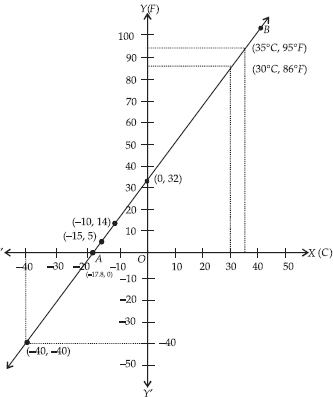Q 15.

Give the geometric representations of y = 3 as an equation
(i) in one variable (ii) in two variables

SOLUTION:

(i) y = 3
because y = 3 is an equation in one variable, i.e. y only.It has a unique solution y = 3 as shown on the number line shown here.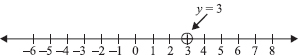The unique solution is a point.
(ii) y = 3
We can write y = 3 as 0 · x + y = 3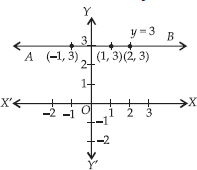Now, when x = 1, y = 3
x = 2, y = 3
x = - 1, y = 3We get the following table: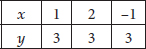Q 16.

Give the geometric representations of 2x + 9 = 0 as an equation
(i) in one variable (ii) in two variables

SOLUTION:

(i) 2x + 9 = 0
We have: 2x + 9 = 0 ⇒ 2x = - 9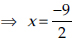which is a linear equation in one variable i.e., x only. Its solution is the point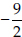on the number line as shown below.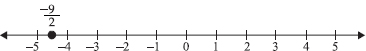(ii) 2x + 9 = 0
We can write 2x + 9 = 0 as 2x + 0 · y + 9 = 0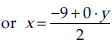When y = 1,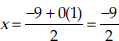When y = 2,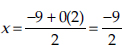When y = 3,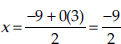When y = 1,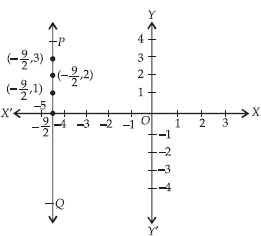Thus, we get the following table: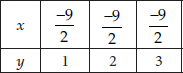Now, plotting the ordered pairs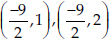and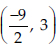on a graph paper and joining them, we get a line PQ as solution of 2x + 9 = 0.